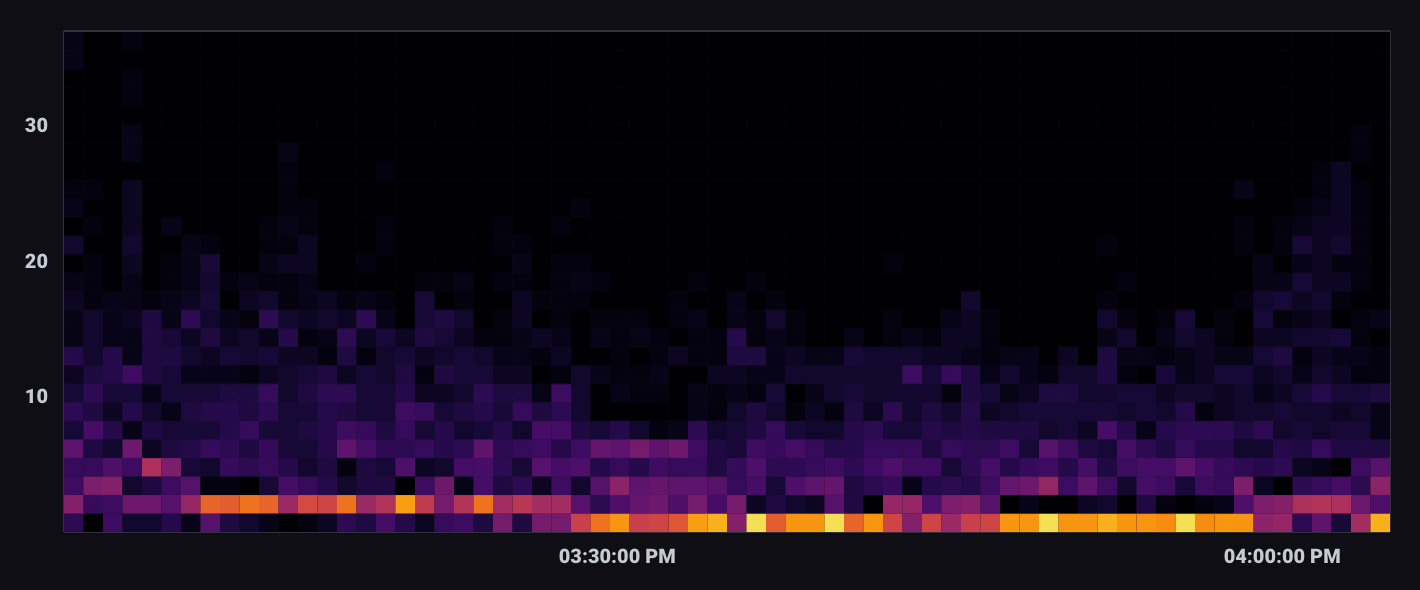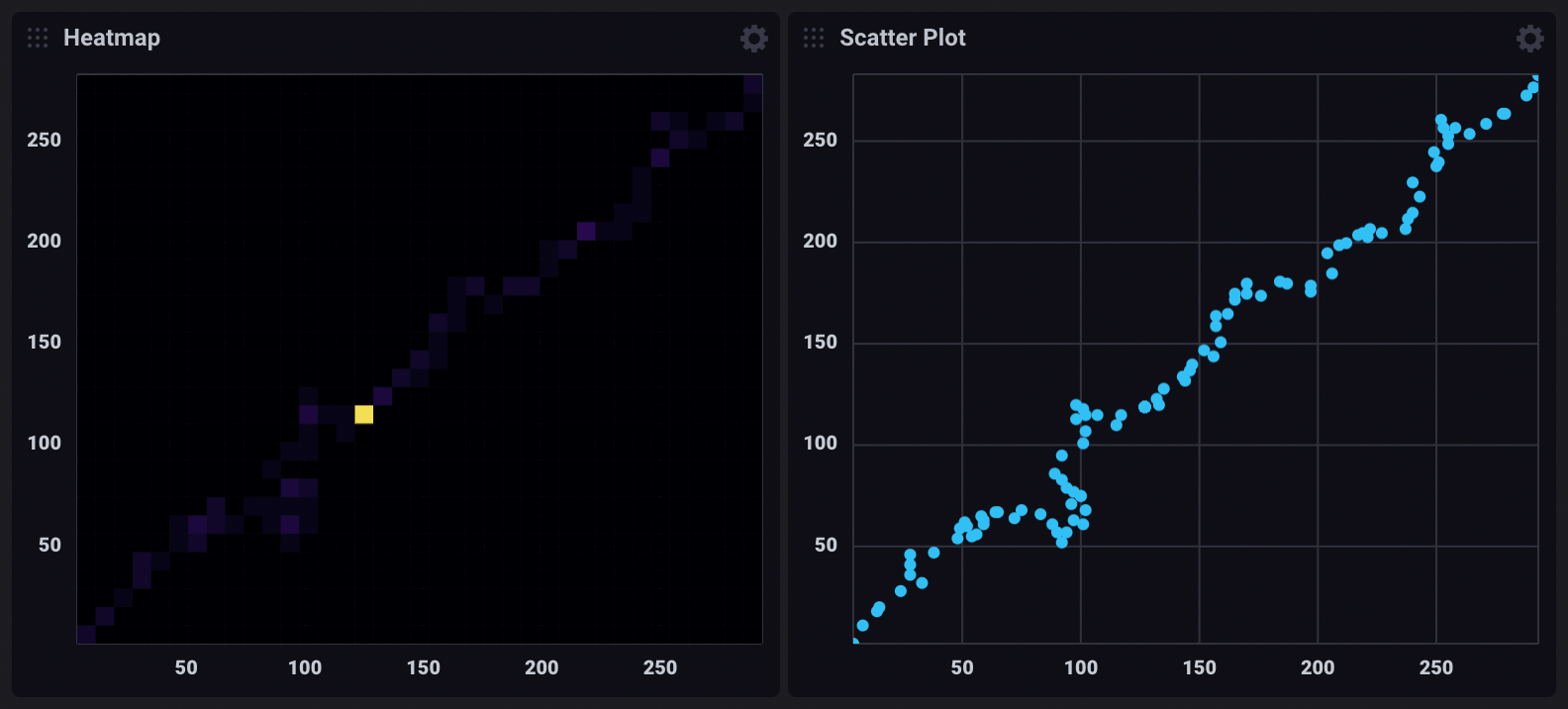# Heatmap visualization

A Heatmap displays the distribution of data on an x and y axes where color represents different concentrations of data points.Select the Heatmap option from the visualization dropdown in the upper left.

## Heatmap behavior

Heatmaps divide data points into “bins” – segments of the visualization with upper and lower bounds for both X and Y axes. The Bin Size option determines the bounds for each bin. The total number of points that fall within a bin determine the its value and color. Warmer or brighter colors represent higher bin values or density of points within the bin.

## Heatmap Controls

To view Heatmap controls, click Customize next to the visualization dropdown.

###### Data
• X Column: Select a column to display on the x-axis.
• Y Column: Select a column to display on the y-axis.
• Time Format: Select the time format. Options include:
• YYYY-MM-DD HH:mm:ss ZZ
• YYYY-MM-DD hh:mm:ss a ZZ
• DD/MM/YYYY HH:mm:ss.sss
• DD/MM/YYYY hh:mm:ss.sss a
• MM/DD/YYYY HH:mm:ss.sss
• MM/DD/YYYY hh:mm:ss.sss a
• YYYY/MM/DD HH:mm:ss
• YYYY/MM/DD hh:mm:ss a
• HH:mm
• hh:mm a
• HH:mm:ss
• hh:mm:ss a
• HH:mm:ss ZZ
• hh:mm:ss a ZZ
• HH:mm:ss.sss
• hh:mm:ss.sss a
• MMMM D, YYYY HH:mm:ss
• MMMM D, YYYY hh:mm:ss a
• dddd, MMMM D, YYYY HH:mm:ss
• dddd, MMMM D, YYYY hh:mm:ss a
###### Options
• Color Scheme: Select a color scheme to use for your heatmap.
• Bin Size: Specify the size of each bin. Default is 10.
###### X Axis
• X Axis Label: Label for the x-axis.
• Generate X-Axis Tick Marks: Select the method to generate x-axis tick marks:
• Auto: Select to automatically generate tick marks.
• Custom: To customize the number of x-axis tick marks, select this option, and then enter the following:
• Total Tick Marks: Enter the total number of ticks to display.
• Start Tick Marks At: Enter the value to start ticks at.
• Tick Mark Interval: Enter the interval in between each tick.
• X Axis Domain: The x-axis value range.
• Auto: Automatically determine the value range based on values in the data set.
• Custom: Manually specify the minimum y-axis value, maximum y-axis value, or range by including both.
• Min: Minimum x-axis value.
• Max: Maximum x-axis value.
###### Y Axis
• Y Axis Label: Label for the y-axis.
• Y Tick Prefix: Prefix to be added to y-value.
• Y Tick Suffix: Suffix to be added to y-value.
• Generate Y-Axis Tick Marks: Select the method to generate y-axis tick marks:
• Auto: Select to automatically generate tick marks.
• Custom: To customize the number of y-axis tick marks, select this option, and then enter the following:
• Total Tick Marks: Enter the total number of ticks to display.
• Start Tick Marks At: Enter the value to start ticks at.
• Tick Mark Interval: Enter the interval in between each tick.
• Y Axis Domain: The y-axis value range.
• Auto: Automatically determine the value range based on values in the data set.
• Custom: Manually specify the minimum y-axis value, maximum y-axis value, or range by including both.
• Min: Minimum y-axis value.
• Max: Maximum y-axis value.
###### Hover Legend
• Orientation: Select the orientation of the legend that appears upon hover:
• Horizontal: Select to display the legend horizontally.
• Vertical: Select to display the legend vertically.
• Opacity: Adjust the legend opacity using the slider.
• Colorize Rows: Select to display legend rows in colors.

## Heatmap examples

### Cross-measurement correlation

The following example explores possible correlation between CPU and Memory usage. It uses data collected with the Telegraf Mem and CPU input plugins.

###### Join CPU and memory usage

The following query joins CPU and memory usage on `_time`. Each row in the output table contains `_value_cpu` and `_value_mem` columns.

``````cpu = from(bucket: "example-bucket")
|> range(start: v.timeRangeStart, stop: v.timeRangeStop)
|> filter(fn: (r) => r._measurement == "cpu" and r._field == "usage_system" and r.cpu == "cpu-total")

mem = from(bucket: "example-bucket")
|> range(start: v.timeRangeStart, stop: v.timeRangeStop)
|> filter(fn: (r) => r._measurement == "mem" and r._field == "used_percent")

join(tables: {cpu: cpu, mem: mem}, on: ["_time"], method: "inner")
``````
###### Use a heatmap to visualize correlation

In the Heatmap visualization controls, `_value_cpu` is selected as the X Column and `_value_mem` is selected as the Y Column. The domain for each axis is also customized to account for the scale difference between column values.## Important notes

### Differences between a heatmap and a scatter plot

Heatmaps and Scatter plots both visualize the distribution of data points on X and Y axes. However, in certain cases, heatmaps provide better visibility into point density.

For example, the dashboard cells below visualize the same query results:The heatmap indicates isolated high point density, which isn’t visible in the scatter plot. In the scatter plot visualization, points that share the same X and Y coordinates appear as a single point.